# Step 1: Volumetric mesh generation

http://sofamacbuilder.lille.inria.fr/defrost/scenes/Tutorial/step1-VolumetricMeshGeneretion/MeshTetraGeneration.py.
(Of course, it is more appealing to test it with SOFA on the virtual machine !)

In order to simulate a mechanical object using Finite Element Modeling (FEM), a discrete version of its volume is required. This volume, referred as volumetric mesh will serve as a domain for FEM computation. The aim of this tutorial step is to explain how to create such a volumetric mesh from a closed surface description made of triangles. Several tools exist for volume meshing such as GID (http://www.gidhome.com/), CGAL (http://www.cgal.org/) or Gmsh (http://geuz.org/gmsh/). In the following, we will use CGAL, as a dedicated plugin is available within the SOFA framework.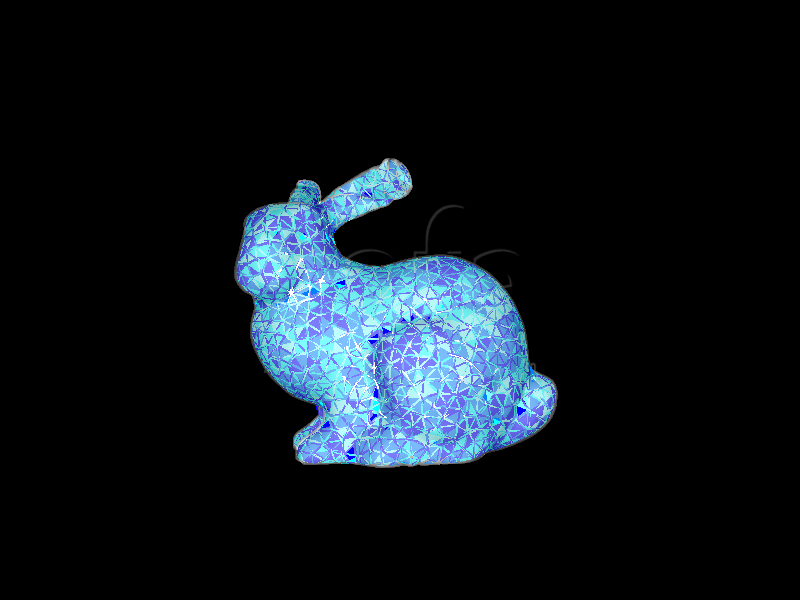## A. First generic example

CGAL creates a 3D volumetric mesh composed of tetrahedra from any closed surface. The SOFA Python file to perform such a procedure should contain at least:

1. The directive that will dynamically link the plugin to SOFA executable:
rootNode.createObject('RequiredPlugin', pluginName='CGALPlugin')

2. We then create a node object that will gather the input and the processing through
node = rootNode.createChild('node')

3. The closed 3D surface which should be described in any 3D format supported by SOFA (OBJ, VTK STL,...) node.createObject('Mesh',name='mesh',filename=path+'bunny.obj')

4. The CGAL method that will process the surface to compute the volumetric mesh:
node.createObject('MeshGenerationFromPolyhedron',inputPoints='@mesh.position', inputTriangles='@mesh.triangles', drawTetras='1')

5. The parameters used for this component are related to the input data: inputPoints, inputTriangles or inputQuads describe the composition of the input mesh

6. The resulting volumetric mesh is then exported in a VTK file by:
node.createObject('Mesh', position='@gen.outputPoints', tetrahedra='@gen.outputTetras')
node.createObject('VTKExporter', filename='bunny', edges='0', tetras='1', exportAtBegin='1')

The first line creates a Mesh object that will be defined by the vertices and the tetrahedra that were computed by CGAL and the second line allows to export the resulting volumetric mesh at the beginning of the SOFA simulation (please note that the .vtu file extension is automatically added at the end of the filename).

By adding the usual SOFA Python directives, the complete scene file is available at the following location on the virtual image hard drive. Running this scene file with SOFA using the Stanford Bunny 3D model (https://graphics.stanford.edu/data/3Dscanrep/ ) leads to the following result: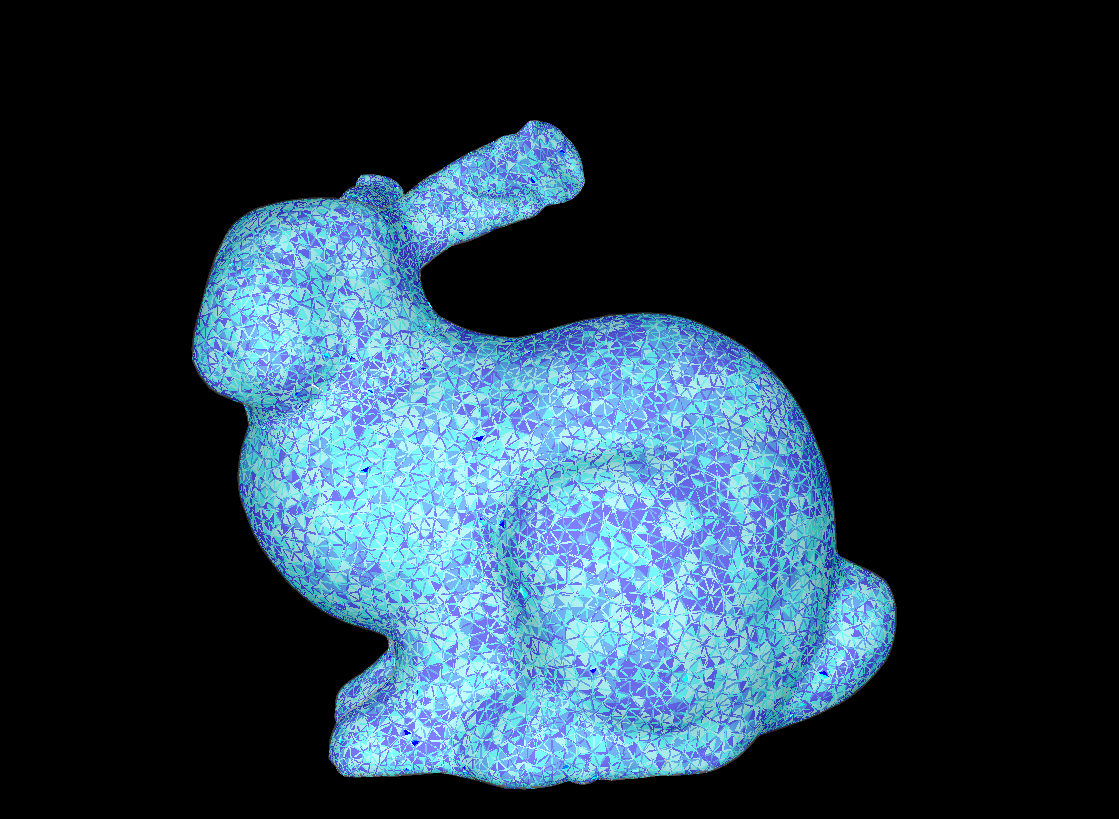Using CGAL with default parameters often creates a very fine mesh composed of many vertices. This may involve a large computational fingerprint that is not compatible with real-time control of the soft robot. The MeshGenerationFromPolyhedron component allows to tune the tetrahedra budget in order to optimize the tradeoff between simulation speed and accuracy. The fine-tuning of these parameters requires some background on Delaunay Triangulation (https://en.wikipedia.org/wiki/Delaunay_triangulation) but some rules of thumb may be provided. There are four parameters that can be modified to change the coarseness of the volumetric mesh:

• facetSize: this parameter provides an upper bound for the radii of the surface Delaunay ball; a larger value may lead to larger tetrahedra.
• facetApproximation: the approximation error between the boundary and the subdivision surface. It provides an upper bound for the distance between the circumcenter of a surface facet and the center of a surface Delaunay ball of this facet.
• cellRatio: This parameter controls the shape of mesh cells. Actually, it is an upper bound for the ratio between the circumradius of a mesh tetrahedron and its shortest edge. There is a theoretical bound for this parameter: the Delaunay refinement process is guaranteed to terminate for values larger than 2.
• cellSize: This parameter controls the size of mesh tetrahedra. It provides an upper bound on the circumradius of the mesh tetrahedra.

Starting from the previous SOFA scene file, we add these four parameters to the MeshGenerationFromPolyhedron component leading to:

 node.createObject('MeshGenerationFromPolyhedron',                                inputPoints='@mesh.position',                                inputTriangles='@mesh.triangles',                                drawTetras='1',facetSize="1.5",                                    facetApproximation="0.15",                                 cellRatio="2", cellSize="1.5")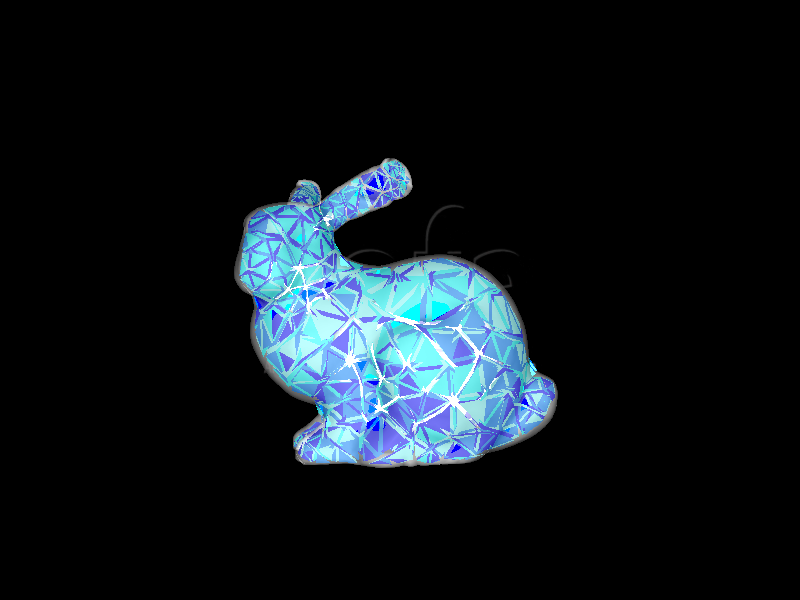Instead of the 26k vertices for the tetrahedral mesh, a coarser mesh is obtained with only 600 vertices. In order to refine the mesh, we can decrease the value of these parameters. For instance, with: node.createObject('MeshGenerationFromPolyhedron',                                 inputPoints='@mesh.position',                                 inputTriangles='@mesh.triangles',                                drawTetras='1',                                facetSize="0.35",                                facetApproximation="0.1",                                 cellRatio="2", cellSize="0.5" )the resulting mesh is composed of 2250 vertices, which provides good accuracy and is within the acceptable range for real-time computations: our models are usually composed of 3-4k vertices.

## C. Gripper model

Given these principles, a volumetric mesh has been created for a finger of the soft gripper. The Python file MeshTetraGeneration.py is launched in SOFA. The resulting volumetric mesh of the finger is composed of ~350 vertices allowing fast computation and enough accuracy given the simplicity of the surface geometry.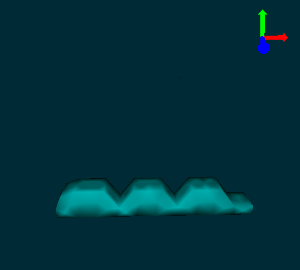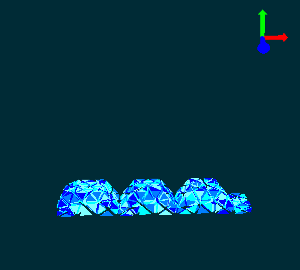Values used to generate this volumetric mesh are:  cellSize=10, facetSize=4, cellRatio=2 and facetApproximation=1.